# Math in Focus Grade 1 Chapter 14 Answer Key Mental Math Strategies

Practice the problems of Math in Focus Grade 1 Workbook Answer Key Chapter 14 Mental Math Strategies to score better marks in the exam.

## Math in Focus Grade 1 Chapter 14 Answer Key Mental Math Strategies

Challenging Practice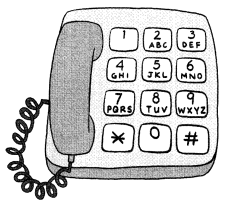You can use a telephone dial to find the value of a word.

Example
YES = 9 + 3 + 7 = 19

Question 1.
Name a three-letter word with a value of 14. _____
Answer: MAT=6+2+6=14 is  a three-letter word with a value of 14

Question 2.
Find a word that has the same value as BOAT. _____
Answer: GUN is a word that has the same value as BOAT
BOAT=2+6+2+8=18
GUN=4+8+6=18

Question 3.
Think of a four-letter word. Find its value. Ask your friend to guess the word.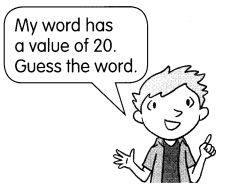Problem Solving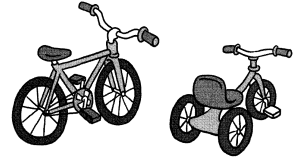There are 6 bicycles and tricycles at Sunshine Day Care Center. There are 16 wheels in all. How many bicycles are there?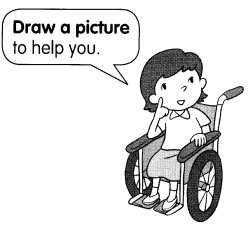Chapter Review/Test
Vocabulary
Choose the correct word.

Question 1.

Question 2.
4 + 4 = 8 is a ____

Concepts and Skills

Question 3.
25 + 4 = ____
adding 25 with 4 we get 29

Question 4.
33 + 4 = ____
adding 33 with 4 we get 37

Question 5.
10 + 28 = ____
Answer: 10 + 28 = 38
adding 10 with 28we get 38

Question 6.
15 + 20 = ____
Answer: 15 + 20 = 35
adding 15 with 20 we get 35

Question 7.
6 + 7 = ___
adding 6 with 7 we get 13

Question 8.
4 + 7 = ____
adding 4 with 7 we get 11

Question 9.
8 – 6 = ____
By removing 6 from 8 we get 2

Question 10.
9 – 7 = ____
By removing 7 from 9 we get 2

Question 11.
16 – 7 = ____
By removing 7 from 16 we get 9

Question 12.
18 – 9 = ____
By removing 9 from 18 we get 9

Question 13.
15 – 8 = ____
By removing 8 from 15 we get 7

Question 14.
14 – 7 = ____
By removing 7 from 14 we get 7

Subtract mentally.

Question 15.
15 – 4 = ______
Answer: 15 – 4 = 11
By removing 4 from 15 we get 11

Question 16.
38 – 6 = ______
By removing 6 from 38 we get 32

Question 17.
21 – 10 = ___
By removing 10 from 21 we get 11

Question 18.
37 – 20 = ___
Answer: 37 – 20 = 17
By removing 20 from 37 we get 17

Problem Solving

Fill in the blanks.

Question 19.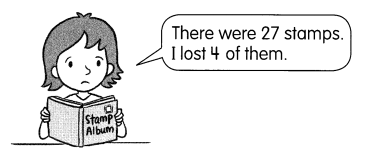How many stamps are left? _____